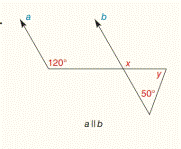Chapter 2.CR, Problem 12CR### Elementary Geometry for College St...

6th Edition
Daniel C. Alexander + 1 other
ISBN: 9781285195698

#### Solutions

Chapter
Section### Elementary Geometry for College St...

6th Edition
Daniel C. Alexander + 1 other
ISBN: 9781285195698
Textbook Problem
11 views

# For Review Exercises 12 to 15 , find the values of x and y .To determine

To find:

The values of x and y.

Explanation

Given:

Line a is parallel to line b.

Figure (1)

Property:

(1) If two parallel lines are cut by a transversal, then a pair of corresponding angles is congruent.

(2) The measure of an exterior angle of a triangle equals the sum of the measures of the two nonadjacent interior angles.

Approach:

Line a is parallel to line b.

120° and x are congruent corresponding angles.

So,

x=120°.

The measure of an exterior angle of a triangle equals the sum of the measures of the two nonadjacent interior angles

### Still sussing out bartleby?

Check out a sample textbook solution.

See a sample solution

#### The Solution to Your Study Problems

Bartleby provides explanations to thousands of textbook problems written by our experts, many with advanced degrees!

Get Started

#### Evaluate the integral, if it exists. 01y(y2+1)5dy

Single Variable Calculus: Early Transcendentals, Volume I

#### 12. Find the minimum value of subject to the condition .

Mathematical Applications for the Management, Life, and Social Sciences

#### For 0

Study Guide for Stewart's Multivariable Calculus, 8th

#### Which number is the best choice for the value of a in the following graph? a) e b) 1 c) 2 d) 3

Study Guide for Stewart's Single Variable Calculus: Early Transcendentals, 8th